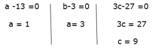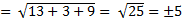## Algebra For SBI PO : Set – 16

D.1-5) In the following questions two equations numbered I and II are given. You have to solve both the equations and Give answer.

1)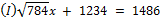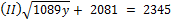a) If x≥y i.e. x is greater than or equal to y

b) If x>y i.e. x is greater than y

c) If x≤y i.e. x is less than or equal to y

d) If x<y i.e. x is less than y

e) If x=y or no relations can be established between x and y

b)

28x = 1486 – 1234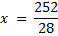x = 9

33y = 2345 – 2081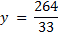y = 8

2)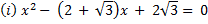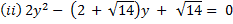a) If x≥y i.e. x is greater than or equal to y

b) If x>y i.e. x is greater than y

c) If x≤y i.e. x is less than or equal to y

d) If x<y i.e. x is less than y

e) If x=y or no relations can be established between x and y

e)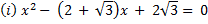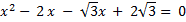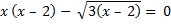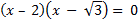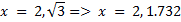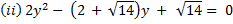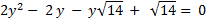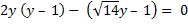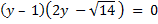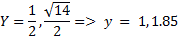3)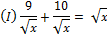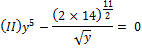a) If x≥y i.e. x is greater than or equal to y

b) If x>y i.e. x is greater than y

c) If x≤y i.e. x is less than or equal to y

d) If x<y i.e. x is less than y

e) If x=y or no relations can be established between x and y

d)x=19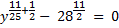y = 28

4)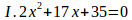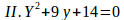a) If x≥y i.e. x is greater than or equal to y

b) If x>y i.e. x is greater than y

c) If x≤y i.e. x is less than or equal to y

d) If x<y i.e. x is less than y

e) If x=y or no relations can be established between x and y

e)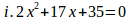70 = 10 , 7

So x = 5 , 3.5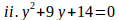14 = 7 , 2

So y = -7 , -2

5)

I) The sum of two number is 5 and their product is 6. Find the value.

II) The length of the rectangle is 1cm less than its width and the area is 30〖cm〗^2. Find the width.

a) If x≥y i.e. x is greater than or equal to y

b) If x>y i.e. x is greater than y

c) If x≤y i.e. x is less than or equal to y

d) If x<y i.e. x is less than y

e) If x=y or no relations can be established between x and y

(e)

I.

x+y = 5                   xy = 6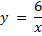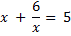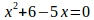x = 2, 3.

II.

width = x

length = x – 1

l × b = 30

(x – 1) x =30

x2 – x – 30 =0

x = 6, -5.

6) The product of two numbers is 594 and the difference of these two numbers is 5. Find the sum of the numbers?

a) 46

b) 39

c) 40

d) 49

e) 59

d)

Let the numbers = x & y

= xy = 594; x – y = 5

(x –y)2 = 25

X2 + y2 – 2xy = 1213

(x + y)2 = x2 + y2 + 2xy

= 1213 + 1189 = 2401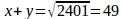7) If P = 999, then the value of p(p2+3p+3) +1 is

a) 1000

b) 999

c) 10

d) 1001

e) 1011

a)

P=999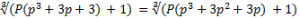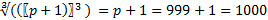8) If2 x-x+1=0 then find the value of x3-2x+1= ?

a) 0

b) 2

c) 1

d) 4

e) -1

b)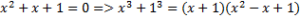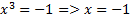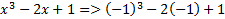=-1+2+1

=2

9) If  x(x+y+z) =  9,  y(x+y+z) =  16  and z(x+y+z) = 144,  then  value  of  x  is

a) 9/5

b) 9/7

c) 9/13

d) 19/13

e) 9/19

(c)

X(x+y+z)+y(x+y+z)+z(x+y+z)=9+16+144

(x+y+z)(x+y+z)=169

Therefore x+y+z=13

Given that x(x+y+z)=9

x=9/13

10)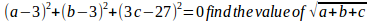a) 5

b) ±5

c) 4

d) ±4

e) 0